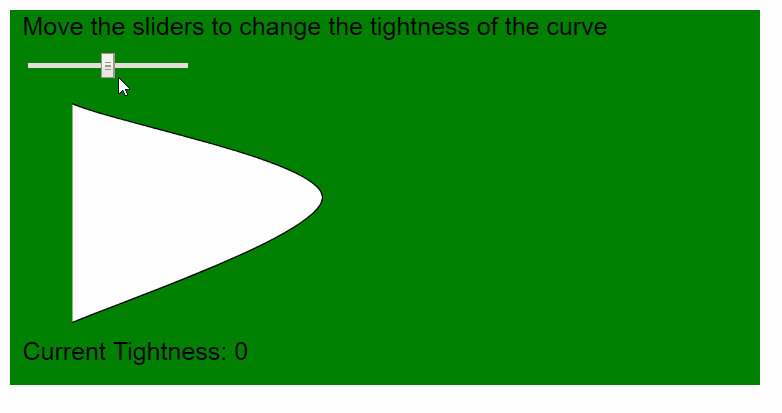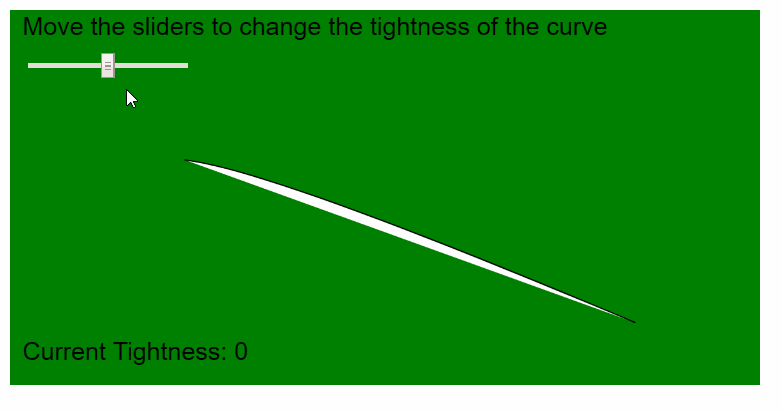# p5.js | curveTightness() Function

• Last Updated : 25 May, 2020

The curveTightness() function in p5.js is used to modify the quality of curves created using the curve() and curveVertex() functions. The tightness parameter is used to define how the curve would fit its vertex points. The default value of tightness for a curve is 0.0 and the value of 1.0 connects all the points with straight lines.
The tightness value can range from -5.0 to 5.0, with the higher values deforming the curve more, while still leaving them recognizable.
Syntax:

```curveTightness( amount )
```

Parameters: This function accepts one parameter as mentioned above and described below:

• amount: It is a number that specifies the amount that the curve would be deformed from its original vertices. This value ranges from -5.0 to 5.0.

Examples below illustrates the curvePoint() function in p5.js:

Example 1:

## javascript

 `function` `setup() {``  ``createCanvas(600, 300);``  ``textSize(20);`` ` `  ``tightnessSlider = createSlider(-5, 5, 0, 0.1);``  ``tightnessSlider.position(20, 40);``}`` ` `function` `draw() {``  ``background(``"green"``);``  ``text(``"Move the sliders to change the tightness of the curve"``, 10, 20);`` ` `  ``// Get the tightness value``  ``tightnessValue = tightnessSlider.value();`` ` `  ``// Set the tightness value``  ``curveTightness(tightnessValue);`` ` `  ``// Draw curve using curveVertex()``  ``beginShape();``  ``curveVertex(20, 50);``  ``curveVertex(50, 75);``  ``curveVertex(250, 150);``  ``curveVertex(50, 250);``  ``curveVertex(20, 250);``  ``endShape();`` ` `  ``text(``"Current Tightness: "` `+ tightnessValue, 10, 280);``}`

Output:Example 2:

## javascript

 `function` `setup() {``  ``createCanvas(600, 300);``  ``textSize(20);`` ` `  ``tightnessSlider = createSlider(-5, 5, 0, 0.1);``  ``tightnessSlider.position(20, 40);``}`` ` `function` `draw() {``  ``background(``"green"``);``  ``text(``"Move the sliders to change the tightness of the curve"``, 10, 20);`` ` `  ``// Get the tightness value``  ``tightnessValue = tightnessSlider.value();`` ` `  ``// Set the tightness value``  ``curveTightness(tightnessValue);`` ` `  ``// Draw curve using curve()``  ``curve(60, 200, 140, 120, 500, 250, 450, 250);`` ` `  ``text(``"Current Tightness: "` `+ tightnessValue, 10, 280);``}`

Output:Online editor: https://editor.p5js.org/
Environment Setup: https://www.geeksforgeeks.org/p5-js-soundfile-object-installation-and-methods/
Reference: https://p5js.org/reference/#/p5/curveTightness

My Personal Notes arrow_drop_up